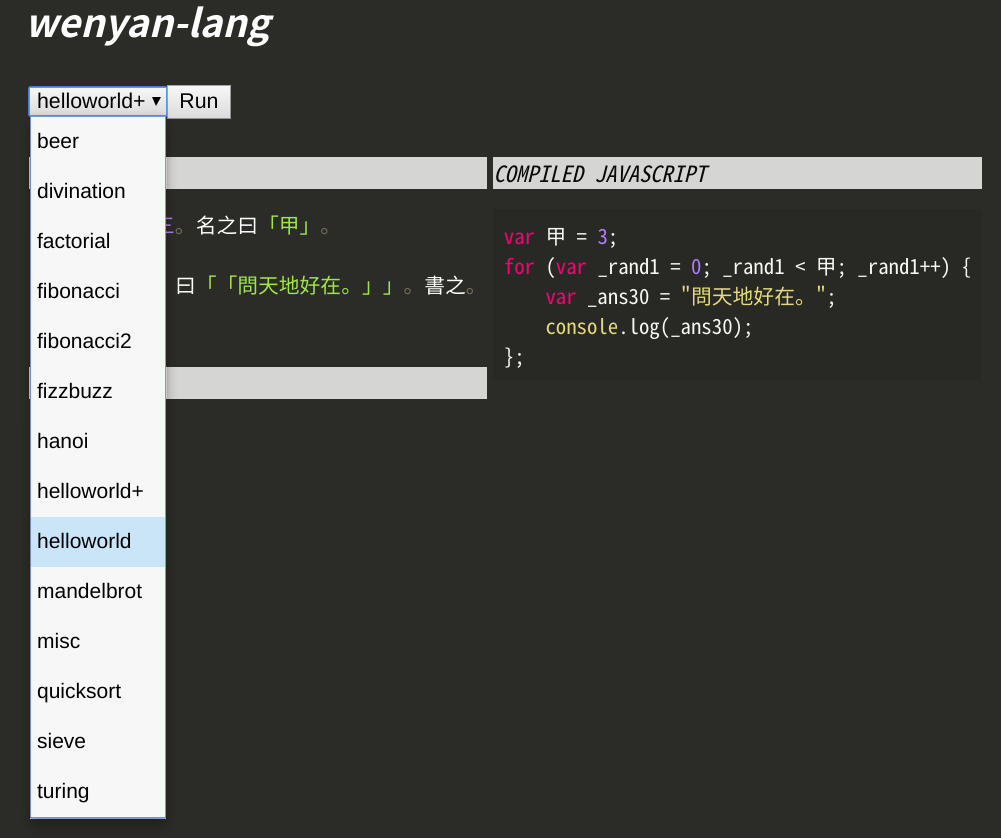# 文言文编程语言来了，可是好像比英文更难写了！```
```吾有一數。曰三。名之曰「甲」。

吾有一言。曰「「問天地好在。」」。書之。

``````

```
var n = 3;
for (var i = 0; i < n; i++) {
console.log("問天地好在。");
}```

```
```問天地好在。

``````

```
`吾有一數曰三名之曰「甲」為是「甲」遍吾有一言曰「「問天地好在」」書之云云````

Helloworld 看上去虽然简单，但是其实作为一门编程语言，这里的语法都有严格的定义：

wenyan JavaScript
`吾有一數。曰三。名之曰「甲」。` `var a = 3;`
`有數五十。名之曰「大衍」。` `var dayan = 50;`
`昔之「甲」者。今「大衍」是也。` `a = dayan;`
`吾有一言。曰「「噫吁戲」」。名之曰「乙」。` `var b = "alas!";`
`吾有一爻。曰陰。名之曰「丙」。` `var c = false;`
`吾有一列。名之曰「丁」。` `var d = [];`
`吾有三數。曰一。曰三。曰五。名之曰「甲」曰「乙」曰「丙」。` `var a=1,b=3,c=5;`

wenyan JavaScript
`若三大於二者。乃得「「想當然耳」」也。` `if (3>2){ return "of course"; }`
`若三不大於五者。乃得「「想當然耳」」。若非。乃得「「怪哉」」也。` `if(3<=5){return "of course"}else{return "no way"}`
`為是百遍。⋯⋯ 云云。` `for (var i = 0; i < 100; i++){ ... }`
`恆為是。⋯⋯ 云云。` `while (true) { ... }`
`凡「天地」中之「人」。⋯⋯ 云云。` `for (var human of world){ ... }`
`乃止。` `break;`

wenyan JavaScript
`加一以二。` `1+2`
`加一於二。` `2+1`
`加一以二。乘其以三。` `(1+2)*3`
`除十以三。所餘幾何。` `10%3`
`減七百五十六以四百三十三。名之曰「甲」。` `var a = 756-433;`
`夫「甲」「乙」中有陽乎。` `a || b`
`夫「甲」「乙」中無陰乎。` `a && b`

wenyan JavaScript
`吾有一列。名之曰「甲」。充「甲」以四。以二。` `var a = []; a.push(4, 2);`
`銜「甲」以「乙」。以「丙」` `a.concat(b).concat(c);`
`夫「甲」之一。` `a`
`夫「甲」之其餘。` `a.slice(1);`
`夫「玫瑰」之「「名」」。` `rose["name"]`
`夫「寶劍」之長。` `sword.length;`

wenyan JavaScript
`吾有一術。名之曰「吸星大法」。是術曰。⋯⋯是謂「吸星大法」之術也。` `function f(){...}`
`吾有一術。名之曰「六脈神劍」。欲行是術。必先得六數。曰「甲」。曰「乙」。曰「丙」。曰「丁」。曰「戊」。曰「己」乃行是術曰。⋯⋯是謂「六脈神劍」之術也。` `function f(a,b,c,d,e,f){...}`
`吾有一術。名之曰「翻倍」。欲行是術。必先得一數。曰「甲」。乃行是術曰。乘「甲」以二。名之曰「乙」。乃得「乙」。是謂「翻倍」之術也。` `function double(a){var b = a * 2; return b;}`